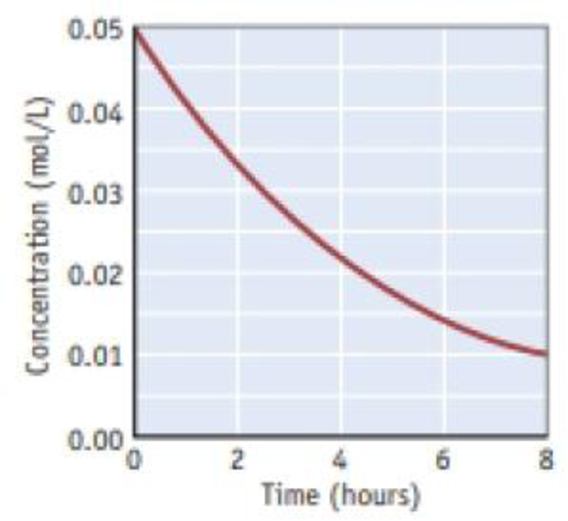Chapter 14.1, Problem 14.1CYU

Chapter
Section
Textbook Problem

Sucrose decomposes to fructose and glucose in acid solution. A plot of the concentration of sucrose as a function of time is given in the margin. What is the rate of change of the sucrose concentration over the first 2 hours? What is the rate of change over the last 2 hours?Concentration versus time for the decomposition of sucrose.

Interpretation Introduction

Interpretation:

The rate of change over the given time intervals has to be given.

Concept Introduction:

The rate of reaction is the quantity of formation of product or the quantity of reactant used per unit time.  The rate of reaction doesn’t depend on the sum of amount of reaction mixture used.

The raise in molar concentration of product of a reaction per unit time or decrease in molarity of reactant per unit time is called rate of reaction and is expressed in units of mol/(L.s) .

The equation that relates the reaction rate to the reactants concentrations that is raised to various powers is called as rate law.

Rate law can be determined by the slow step or otherwise called as rate-determining step.

Rate constant is defined as proportionality constant in the connection between rate and concentrations.

Explanation

The rate of the reaction is calculated as,

Rateofdecompositionofdye  =  -change in [dye]change in time  =  -Δ[dye]ΔtAtt = 0, the concentration of dye  =  0.05 mol/LAt t = 2 hrs, the concentration of dye  =  0.034 mol/LChange in concentration  = cfinal-cinitial = (0.05mol/L)-(0.034mol/L)                                                                  = 0.016 mol/LRateofdecompositionofdye   -Δ[dye]Δt = -(0

Still sussing out bartleby?

Check out a sample textbook solution.

See a sample solution

The Solution to Your Study Problems

Bartleby provides explanations to thousands of textbook problems written by our experts, many with advanced degrees!

Get Started

To overload a muscle is never productive. T F

Nutrition: Concepts and Controversies - Standalone book (MindTap Course List)

What are the largest known structures in the Universe?

Horizons: Exploring the Universe (MindTap Course List)

What is the composition of air?

Oceanography: An Invitation To Marine Science, Loose-leaf Versin